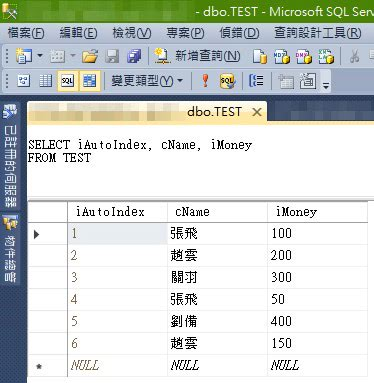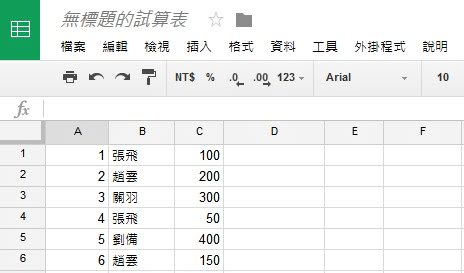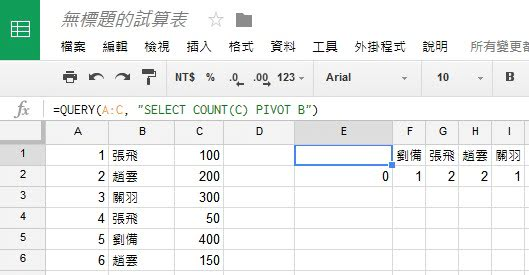﻿## 先來談談SQL的做法SQL指令如下：

``````SELECT cName, COUNT(cName) AS count
FROM TEST
GROUP BY cName
````````````=QUERY(A:C, "SELECT B, COUNT(B) WHERE B<>'' GROUP BY B ORDER BY COUNT(B) DESC")
``````

``````=QUERY(A:C, "SELECT B, COUNT(C) WHERE B<>'' GROUP BY B ORDER BY COUNT(C) DESC")
````````````=QUERY(A:C, "SELECT COUNT(C) PIVOT B")